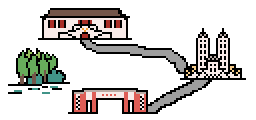# 4552. Short PathOur school is composed of a building set $V$ and a road set $E$. Each road in $E$ can be written as a tuple $(i,j,w)$, which means the road connects two buildings $i$ and $j$ and the length of the road is $w(w>0)$.

A path between two buildings $i$ and $j$ can be defined as a sequence of roads $(i,v_1,w_1),(v_1,v_2,w_2),(v_2,v_3,w_3)\cdots (v_k,j,w_{k+1})$(or just only one road $(i,j,w)$). And the length of a path is the sum of the lengths of the roads in the path.

The shortest distance between two buildings $i$ and $j$ is the minimum length of the paths between $i$ and $j$.

In this problem, given is the number of buildings in our school and the exact shortest distances between any two buildings.

You should construct the roads in the school and make the given shortest distances satisfied. Moreover, because of the poverty of our school, we want you to minimize the sum of the lengths of roads in school and output the minimum sum.

If there is no construction that satisfies the given shortest distances, you should output -1.

### 输入格式

The first line contains an integer $n$, indicating the number of the buildings in the school.

The next $n$ lines, each line contains $n$ integers. The $j$-th integer of the $i$-th line is $a_{ij}$, indicating the shortest distance between building $i$ and building $j$.

### 输出格式

Outputs the minimum total length of the roads.

If a legal way to build the roads does not exist, output -1.

### 样例

Input
3
0 1 3
1 0 2
3 2 0

Output
3

Input
3
0 1 4
1 0 2
4 2 0

Output
-1


### 提示

$1 \leq n \leq 500$，$1 \le a_{ij} \le 10^9~(i \not= j)$，$a_{ii} = 0$，$a_{ij} = a_{ji}$

Sample 1 Explanation:
Build roads: $(1,2,1)$ and $(2,3,2)$

97 人解决，142 人已尝试。

104 份提交通过，共有 502 份提交。

4.1 EMB 奖励。